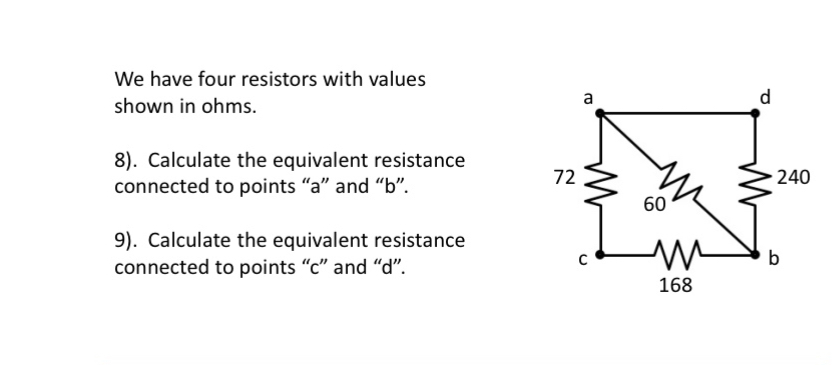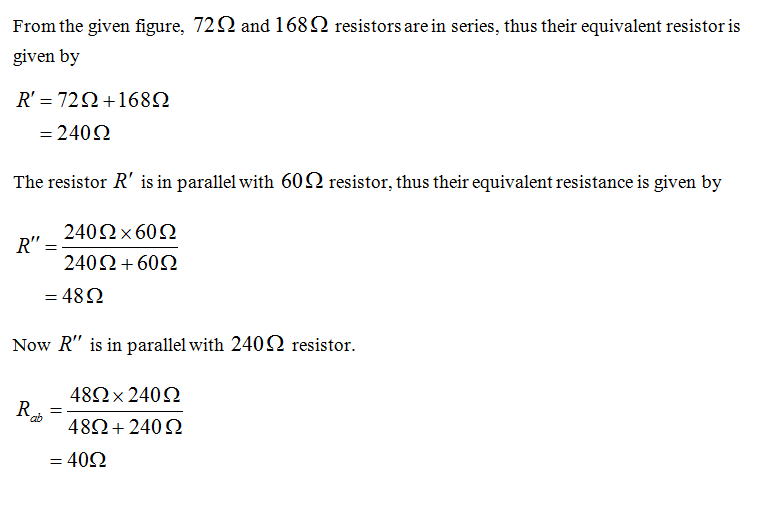# We have four resistors with valuesashown in ohms.8). Calculate the equivalent resistanceconnected to points "a" and "b".72240609). Calculate the equivalent resistanceconnected to points "c" and “d".b168

Question
2 viewshelp_outlineImage TranscriptioncloseWe have four resistors with values a shown in ohms. 8). Calculate the equivalent resistance connected to points "a" and "b". 72 240 60 9). Calculate the equivalent resistance connected to points "c" and “d". b 168 fullscreen
check_circle

Step 1...

### Want to see the full answer?

See Solution

#### Want to see this answer and more?

Solutions are written by subject experts who are available 24/7. Questions are typically answered within 1 hour.*

See Solution
*Response times may vary by subject and question.
Tagged in